Notebook

# Event Study with EventVestor's Share Buybacks¶

While there are a few different ways you could analyze a financial event, two stand out to be the easiest and most widely used strategies. The first is to create an algorithm based off it and look at how it does compared to the SPY (for those who haven’t seen it, I highly suggest taking a look here first). The second is to conduct an actual event study looking at the impact of an event on a stock’s price. This impact is measured through something abnormal returns. Abnormal returns are simply the returns that were caused by an event compared to what the returns would normally be. Understanding the abnormal returns around an event help to understand whether the event can be a profitable, alpha-generating signal for trading. So while my algorithm (mentioned in the post above) generates more than 2 times the return of the SPY, I’m going to take a closer look at the event to maybe understand HOW and WHY my algorithm might be doing that. In the process, you’re going to learn how to conduct an event study and replicate the results for your own datasets. I promise you’ll learn a lot and will walk away feeling a bit more comfortable with the new research platform.

# Conducting our event study¶

Before I take you into all the code, I’m going to layout the structure of this notebook so you have a general sense of where I’m going:

• First, I’m going to spend some time loading in the data and writing a few helper functions that I’m going to use repeatedly throughout my notebook.
• Next, I’ll be looking first at the average cumulative returns of all stocks around the time that an event was announced (you’ll see what I mean) and the average cumulative abnormal returns of all stocks in the same time period. I look at both in order to compare how the event does independently of a benchmark and how it’d fare if compared simply against the SPY.
• Lastly, I’m going to look at the volatility of the average returns that I found in the previous step, just to get a sense of the noise level of this event.
In :
"""
Step One: Load our imports and data
"""

import numpy as np
import pandas as pd
import seaborn as sns
import matplotlib.pyplot as pyplot
from datetime import timedelta

min_date = pd.to_datetime("12/31/2006")

#: EventVestor data
ev_data_raw = local_csv('event_vestor_data_complete.csv')

#: Converting our Dates into a Series of Datetimes so we can do some date logic easily
ev_data_raw['event_date'] = pd.to_datetime(ev_data_raw['event_date'])
ev_data_raw = ev_data_raw[(ev_data_raw['event_date'] > min_date)]

ev_data = ev_data[(ev_data.index > min_date)]
ev_data = ev_data[(ev_data['symbol'] != 0)]

"""
Get just the closing and open prices for our symbols
"""

ev_symbols = symbols(np.append(ev_data_raw['symbol'].unique(), ['SPY']), handle_missing='ignore')

data = get_pricing(ev_symbols,
fields=['close_price'],
start_date='2007-01-01',
end_date = '2015-02-01')


By now, I've loaded in essentialy two pieces of data:

• EventVestor's Share Buyback's Announcement Data
• Stock pricing data for all valid tickers (close price)

So since I have the close price for all these securities from 2007-2015, what I'm going to do is look at a band around each ticker's share buyback announcement date and track the movement of its stock price. By band I mean a specific timeframe around the event (which I'm specifiying as t=0).

In :
"""
Step Two: Creating some helper functions to find our open prices and close prices
"""

def get_close_price(data, sid, current_date, day_number):
#: If we're looking at day 0 just return the indexed date
if day_number == 0:
return data['close_price'].ix[current_date][sid]
#: Find the close price day_number away from the current_date
else:
#: If the close price is too far ahead, just get the last available
total_date_index_length = len(data['close_price'].index)
#: Find the closest date to the target date
date_index = data['close_price'].index.searchsorted(current_date + timedelta(day_number))
#: If the closest date is too far ahead, reset to the latest date possible
date_index = total_date_index_length - 1 if date_index >= total_date_index_length else date_index
#: Use the index to return a close price that matches
return data['close_price'].iloc[date_index][sid]

def get_first_price(data, starting_point, sid, date):
starting_day = date - timedelta(starting_point)
date_index = data['close_price'].index.searchsorted(starting_day)
return data['close_price'].iloc[date_index][sid]

def remove_outliers(returns, num_std_devs):
return returns[~((returns-returns.mean()).abs()>num_std_devs*returns.std())]

def get_returns(data, starting_point, sid, date, day_num):
#: Get stock prices
first_price = get_first_price(data, starting_point, sid, date)
close_price = get_close_price(data, sid, date, day_num)

#: Calculate returns
ret = (close_price - first_price)/(first_price + 0.0)
return ret


The get_close_price function is definitely a little tricky to tackle at first. The basic gist of it is that Pandas provides a very helpful method called searchsorted that allows you to look at the dates of an index and find the closest date BUT it returns an index number, not the actual index. Finally, I take that index number and then use .iloc to get the close price that belongs to the number.

In :
"""
Step Three: Calculate average cumulative returns
"""

#: Dictionaries that I'm going to be storing calculated data in
all_returns = {}
all_std_devs = {}
total_sample_size = {}

#: Create our range of day_numbers that will be used to calculate returns
starting_point = 30
#: Looking from -starting_point till +starting_point which creates our timeframe band
day_numbers = [i for i in range(-starting_point, starting_point)]

for day_num in day_numbers:

#: Reset our returns and sample size each iteration
returns = []
sample_size = 0

#: Get the return compared to t=0
for date, row in ev_data.iterrows():
sid = row.symbol

#: Make sure that data exists for the dates
if date not in data['close_price'].index or sid not in data['close_price'].columns:
continue

returns.append(get_returns(data, starting_point, sid, date, day_num))
sample_size += 1

#: Drop any Nans, remove outliers, find outliers and aggregate returns and std dev
returns = pd.Series(returns).dropna()
returns = remove_outliers(returns, 2)
all_returns[day_num] = np.average(returns)
all_std_devs[day_num] = np.std(returns)
total_sample_size[day_num] = sample_size

#: Take all the returns, stds, and sample sizes that I got and put that into a Series
all_returns = pd.Series(all_returns)
all_std_devs = pd.Series(all_std_devs)
N = np.average(pd.Series(total_sample_size))

In :
"""
Step Four: Plotting our event study graph
"""

xticks = [d for d in day_numbers if d%2 == 0]
all_returns.plot(xticks=xticks, label="N=%s" % N)

pyplot.grid(b=None, which=u'major', axis=u'y')
pyplot.title("Cumulative Return from Share Buyback Announcements before and after event")
pyplot.xlabel("Window Length (t)")
pyplot.legend()
pyplot.ylabel("Cumulative Return (r)")

Out:
<matplotlib.text.Text at 0x7fb0e0918b90>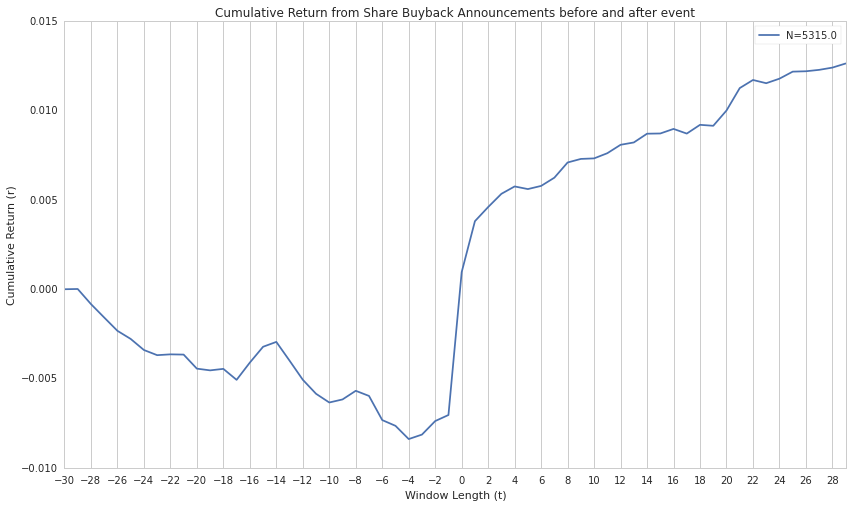It's clear that the upspike exists around t=0 with a 1% upspike from the end of closing on t=-1 and a 1% drift from t=0 till t=30.

Interestingly, you'll see that most of the upspike begins at the end of trading on t=-1 and ends on the trading day of t=0. This is pretty common for a big event like this since the fastest hits on an event will happen immediately after the event is announced. So in this case, the buyback announcement might've occured after market close on t=-1 and by the time it reaches market open on t=0, most of the alpha from that is gone. That's where the drift comes in.

Immediately after the 1% spike in average return, there's this gentle drift upwards (also ~1%). This is what retail investors cash in on. This is what YOU can trade on.

So by now you might be saying, "Okay! Then let me trade on it!" Well it isn't as simple as that. Remember that uptill now, I've only shown you cumulative returns, not cumulative abnormal returns.

By definition, abnormal returns are the difference between the actual returns of the security and expected return. In this case, I'm measuring how much of the returns are "triggered" by an event (Share buybacks announcement). Here's the simplest version of how to calculate abnormal returns:

AR = Stock Return - (Beta*Market Return)

In :
"""
Comparing with the benchmark's cumulative returns
"""

all_returns = {}
benchmark_returns = {}

#: Create our range of day_numbers that will be used to calculate returns
starting_point = 30
day_numbers = [i for i in range(-starting_point, starting_point)]

for day_num in day_numbers:

#: Reset our returns and sample size each iteration
returns = []
b_returns = []
sample_size = 0

#: Get the return compared to t=0
for date, row in ev_data.iterrows():
sid = row.sid

#: Make sure that data exists for the dates
if date not in data['close_price'].index or sid not in data['close_price'].columns:
continue

returns.append(get_returns(data, starting_point, sid, date, day_num))
#: 8554 is the sid for the benchmark
b_returns.append(get_returns(data, starting_point, 8554, date, day_num))

#: Drop any Nans, remove outliers, find outliers and aggregate returns and std dev
all_returns[day_num] = np.average(remove_outliers(pd.Series(returns).dropna(), 2))
benchmark_returns[day_num] = np.average(pd.Series(b_returns).dropna())

#: Plot
xticks = [d for d in day_numbers if d%2 == 0]
all_returns = pd.Series(all_returns)
benchmark_returns = pd.Series(benchmark_returns)
benchmark_returns.plot(xticks=xticks, label='Benchmark')

pyplot.title("Comparing the benchmark's average returns around that time to PSBAD")
pyplot.ylabel("% Cumulative Return")
pyplot.xlabel("Time Window")
pyplot.legend()
pyplot.grid(b=None, which=u'major', axis=u'y')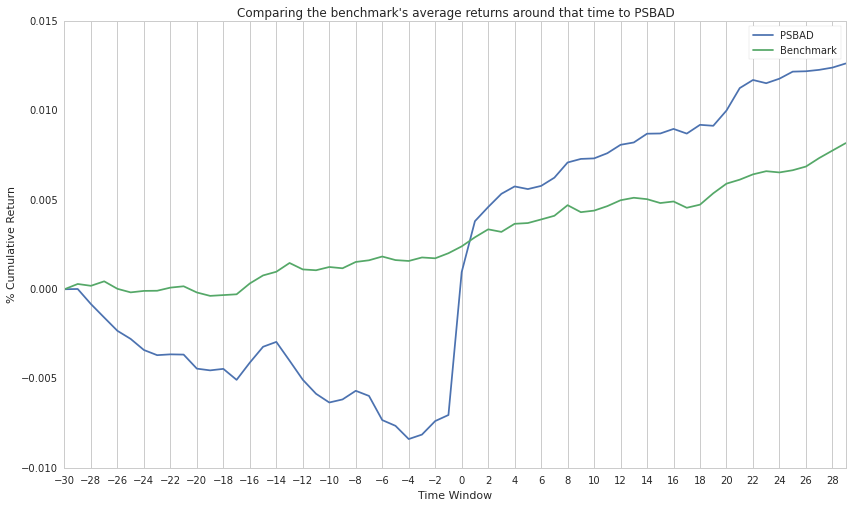In :
"""
Now plotting strictly the abnormal returns using a rolling 30 day beta
"""

def calc_beta(stock, benchmark, price_history):
"""
Calculate our beta amounts for each security
"""
stock_prices = price_history[stock].pct_change().dropna()
bench_prices = price_history[benchmark].pct_change().dropna()
aligned_prices = bench_prices.align(stock_prices,join='inner')
bench_prices = aligned_prices
stock_prices = aligned_prices
bench_prices = np.array( bench_prices.values )
stock_prices = np.array( stock_prices.values )
bench_prices = np.reshape(bench_prices,len(bench_prices))
stock_prices = np.reshape(stock_prices,len(stock_prices))
if len(stock_prices) == 0:
return None
m, b = np.polyfit(bench_prices, stock_prices, 1)
return m

#: Create our range of day_numbers that will be used to calculate returns
ab_all_returns = {}
ab_volatility = {}

starting_point = 30
day_numbers = [i for i in range(-starting_point, starting_point)]

for day_num in day_numbers:

#: Reset our returns and sample size each iteration
returns = []
b_returns = []
sample_size = 0

#: Get the return compared to t=0
for date, row in ev_data.iterrows():
sid = row.sid

#: Make sure that data exists for the dates
if date not in data['close_price'].index or sid not in data['close_price'].columns:
continue

ret = get_returns(data, starting_point, sid, date, day_num)
b_ret = get_returns(data, starting_point, 8554, date, day_num)

"""
Calculate beta by getting the last X days of data
1. Create a DataFrame containing the data for the necessary sids within that time frame
2. Pass that DataFrame into our calc_beta function in order to spit out a beta
"""
history_index = data['close_price'].index.searchsorted(date)
history_index_start = max([history_index - starting_point, 0])
price_history = data['close_price'].iloc[history_index_start:history_index][[sid, 8554]]
beta = calc_beta(sid, 8554, price_history)
if beta is None:
continue

#: Calculate abnormal returns
abnormal_return = ret - (beta*b_ret)
returns.append(abnormal_return)

#: Drop any Nans, remove outliers, find outliers and aggregate returns and std dev
returns = pd.Series(returns).dropna()
returns = remove_outliers(returns, 2)

ab_volatility[day_num] = np.std(returns)
ab_all_returns[day_num] = np.average(returns)

In :
"""
Plotting cumulative abnormal returns
"""
xticks = [d for d in day_numbers if d%2 == 0]
ab_all_returns = pd.Series(ab_all_returns)
ab_all_returns.plot(xticks=xticks, label="Abnormal Average Cumulative")
all_returns.plot(xticks=xticks, label="Simple Average Cumulative")

pyplot.axhline(y=ab_all_returns.ix, linestyle='--', color='black', alpha=.3, label='Drift')
pyplot.axhline(y=ab_all_returns.max(), linestyle='--', color='black', alpha=.3)
pyplot.title("Cumulative Abnormal Returns versus Cumulative Returns")
pyplot.ylabel("% Cumulative Return")
pyplot.xlabel("Time Window")
pyplot.grid(b=None, which=u'major', axis=u'y')
pyplot.legend()

Out:
<matplotlib.legend.Legend at 0x7fb0e35501d0>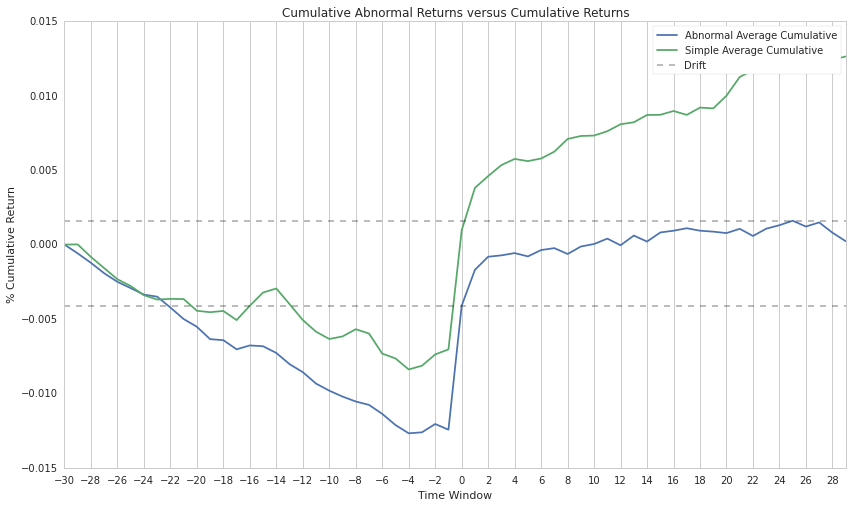Just a few things to note, you can see that the same general pattern stays the same. The quick upspike in price directly after the announcement and a general positive drift a few days after. The main difference here is that in the case of the cumulative abnormal returns (where you are comparing against the SPY), there's plateau and even a movement downwards towards the end of the time frame. This is why in the algorithm, the holding period was set to 7 days rather than something longer or shorter in order to maximize capturing the drift after the buyback announcement.

What's also important to note here is that it's important to focus on the movement of the stock price not necessarily it's absolute numbers! E.g. You obtain about a .5% abnormal drift after the buybacks announcement (on average).

A buyback can affect companys differently and it really does depend on investor perception. For example, if investors perceive that a buyback comes from a good root (e.g. internal executives really do believe the company is undervalued), then the price can react positively. But there's the inverse where the buyback could be perceived to be a negative and the price can react downwards instead. So in an effort to capture just how differently the stock price can react, I'm going to look at the volatility of returns after the event.

In :
"""
Plotting the same graph but with error bars
"""

all_std_devs.ix[:-1] = 0
pyplot.errorbar(all_returns.index, all_returns, xerr=0, yerr=all_std_devs, label="N=%s" % N)
pyplot.grid(b=None, which=u'major', axis=u'y')
pyplot.title("Cumulative Return from Share Buyback Announcements before and after event with error")
pyplot.xlabel("Window Length (t)")
pyplot.ylabel("Cumulative Return (r)")
pyplot.legend()
pyplot.show()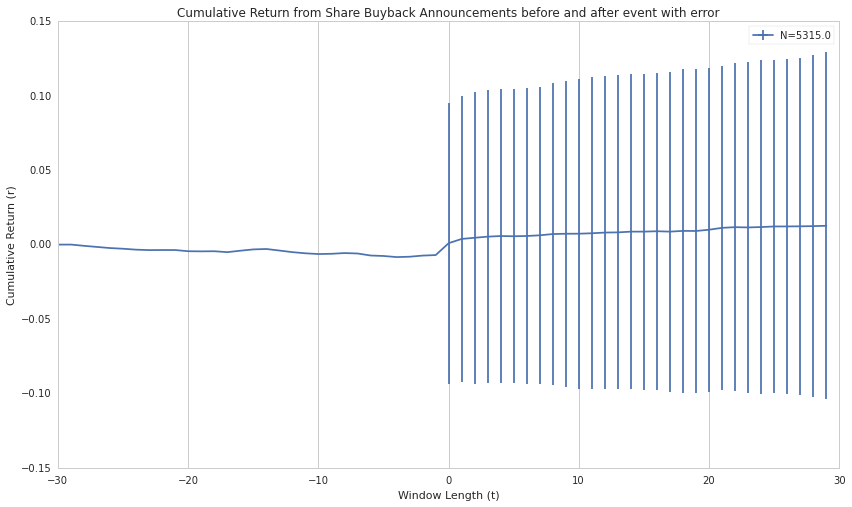In :
"""
Capturing volatility of abnormal returns
"""
ab_volatility = pd.Series(ab_volatility)
ab_all_returns = pd.Series(ab_all_returns)
ab_volatility.ix[:-1] = 0
pyplot.errorbar(ab_all_returns.index, ab_all_returns, xerr=0, yerr=ab_volatility, label="N=%s" % N)
pyplot.grid(b=None, which=u'major', axis=u'y')
pyplot.title("Cumulative Abnormal Returns from Share Buyback Announcements before and after event with error")
pyplot.xlabel("Window Length (t)")
pyplot.ylabel("Cumulative Return (r)")
pyplot.legend()
pyplot.show()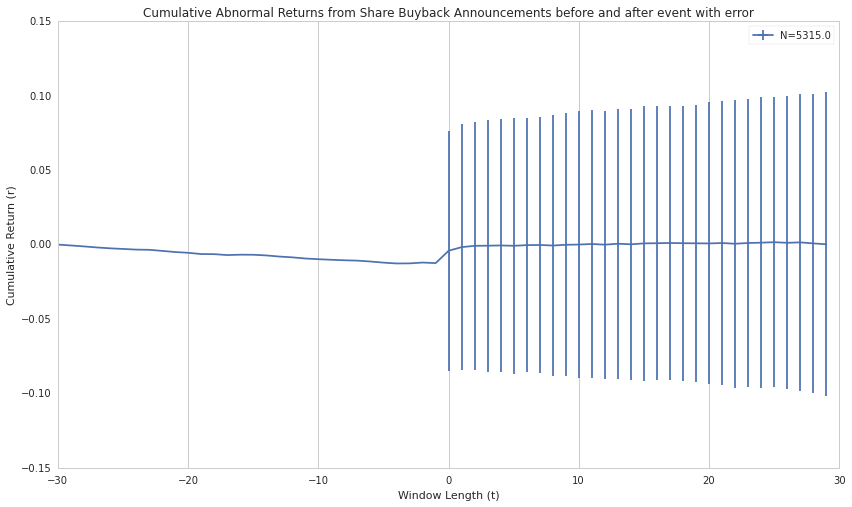So as you can see, the volatility is quite big in either direction. This implies, as is true for many events, that there's a bit of noise in there. It's something that you'll have to take into account when creating an algorithm based off this event.

One thing you might helpful is to narrow down the scope of your data. In previous algorithms, I've done that with percent of shares bought back or the specific sector that a stock belongs to (e.g. Technology or Finance). Here, I'm going to be doing something a little different and actually compare year over year to see whether or not the time effects the volatility of a share buyback.

In :
"""
Comparing Abnormal Buybacks of 2009 versus 2013
"""

"""
Step One: Load our imports and data for 2013
"""

min_date = pd.to_datetime("12/31/2012")
max_date = pd.to_datetime("01/01/2014")
ev_data_13 = ev_data_13[(ev_data_13.index > min_date)]
ev_data_13 = ev_data_13[(ev_data_13.index < max_date)]
ev_data_13 = ev_data_13[(ev_data_13['symbol'] != 0)]

"""
Step Two: Load our imports and data for 2009
"""

min_date = pd.to_datetime("12/31/2008")
max_date = pd.to_datetime("01/01/2010")
ev_data_9 = ev_data_9[(ev_data_9.index > min_date)]
ev_data_9 = ev_data_9[(ev_data_9.index < max_date)]
ev_data_9 = ev_data_9[(ev_data_9['symbol'] != 0)]

In :
"""
Step Three: Going through our same volatility and return calculations from above and finding
answers for different types of datasets
"""
starting_point = 30
day_numbers = [i for i in range(-starting_point, starting_point)]

def get_volatility_and_all_returns(ev_data_type, ab_volatility, ab_all_returns):
for day_num in day_numbers:

#: Reset our returns and sample size each iteration
returns = []
b_returns = []
sample_size = 0

#: Get the return compared to t=0
for date, row in ev_data_type.iterrows():
sid = row.symbol

#: Make sure that data exists for the dates
if date not in data['close_price'].index or sid not in data['close_price'].columns:
continue

ret = get_returns(data, starting_point, sid, date, day_num)
b_ret = get_returns(data, starting_point, 8554, date, day_num)

"""
Calculate beta by getting the last X days of data
1. Create a DataFrame containing the data for the necessary sids within that time frame
2. Pass that DataFrame into our calc_beta function in order to spit out a beta
"""
history_index = data['close_price'].index.searchsorted(date)
history_index_start = max([history_index - starting_point, 0])
price_history = data['close_price'].iloc[history_index_start:history_index][[sid, 8554]]
beta = calc_beta(sid, 8554, price_history)
if beta is None:
continue

#: Calculate abnormal returns
abnormal_return = ret - (beta*b_ret)
returns.append(abnormal_return)

#: Drop any Nans, remove outliers, find outliers and aggregate returns and std dev
returns = pd.Series(returns).dropna()
returns = remove_outliers(returns, 2)

ab_volatility[day_num] = np.std(returns)
ab_all_returns[day_num] = np.average(returns)
return ab_volatility, ab_all_returns

#: Find volatility and return levels for both years
ab_volatility_9, ab_all_returns_9 = get_volatility_and_all_returns(ev_data_9, {}, {})
ab_volatility_13, ab_all_returns_13 = get_volatility_and_all_returns(ev_data_13, {}, {})

In :
"""
Capturing volatility of abnormal returns from 2009 versus 2014
"""
ab_volatility_9 = pd.Series(ab_volatility_9)
ab_all_returns_9 = pd.Series(ab_all_returns_9)
ab_volatility_9.ix[:-1] = 0
ab_volatility_13 = pd.Series(ab_volatility_13)
ab_all_returns_13 = pd.Series(ab_all_returns_13)
ab_volatility_13.ix[:-1] = 0

pyplot.errorbar(ab_all_returns_9.index, ab_all_returns_9, xerr=0, yerr=ab_volatility_9, label="2009", alpha=.2)
pyplot.errorbar(ab_all_returns_13.index, ab_all_returns_13, xerr=0, yerr=ab_volatility_13, label="2013")

pyplot.grid(b=None, which=u'major', axis=u'y')
pyplot.title("Cumulative Abnormal Returns")
pyplot.xlabel("Window Length (t)")
pyplot.ylabel("Cumulative Return (r)")
pyplot.legend()
pyplot.show()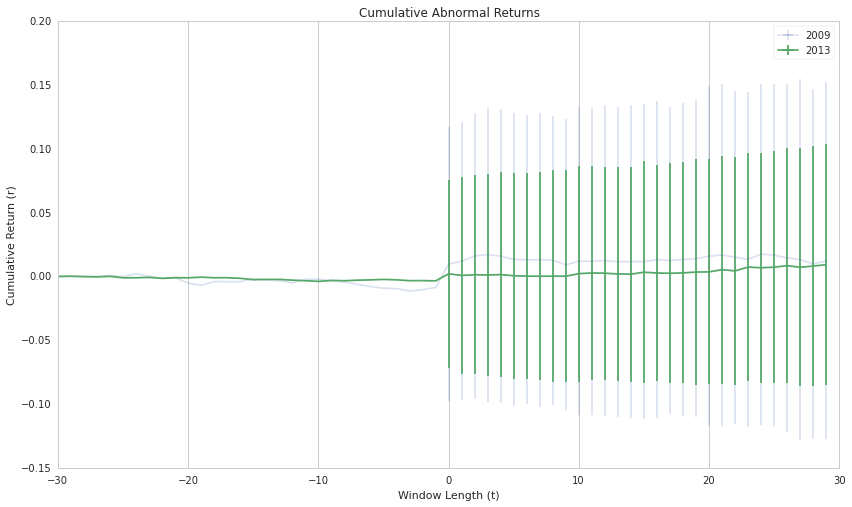Perhaps you could reduce that by looking at only big cap companies, or those with a PE ratio greater than 10 but no less than 20. Or maybe you even want to take out all technology stocks, finance stocks. In my case, I used percent of shares bought back as a way to filter down my securities. If you're curious on how I did that, check out the the first notebook that shows you some of the things I've just mentioned.

# Summary¶

Share buybacks announcements seem to have a significant impact on stock prices immediately following the event as well as in the days that follow (allowing retail investors to catch the drift). However, dependent on the year that you're trading on, the volatility of returns differs significantly with years like 2009 being a lot more volatile than 2013. As always, the data is available through Event Vestor (http://bit.ly/1zGbhXM) if you'd like to sample and test it out for yourselves.

And that concludes this notebook. Most of the code here is easily replicated and as always, the Quantopian team is here to answer any questions you might have.